Home > CC4 > Chapter 5 > Lesson 5.1.3 > Problem5-35

5-35.
1.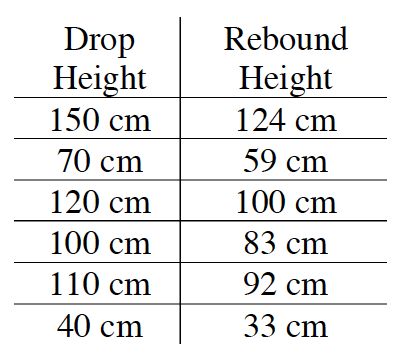DeShawna and her team gathered data for their ball and recorded it in the table shown at right. Homework Help ✎

1. What is the rebound ratio for their ball?

2. Predict how high DeShawna’s ball will rebound if it is dropped from 275 cm. Look at the precision of DeShawna’s measurements in the table. Round your calculation to a reasonable number of decimal places.

3. Suppose the ball is dropped and you notice that its rebound height is 60 cm. From what height was the ball dropped? Use an appropriate precision for your answer.

4. Suppose the ball is dropped from a window 200 meters up the Empire State Building. What would you predict the rebound height to be after the first bounce?

5. How high would the ball in part (d) rebound after the second bounce? After the third bounce?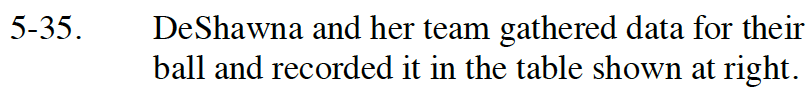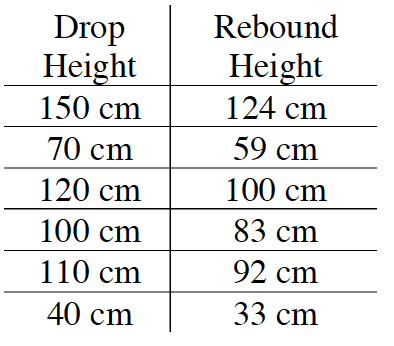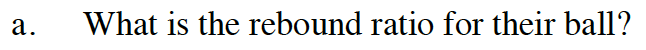Divide a rebound height by a drop height.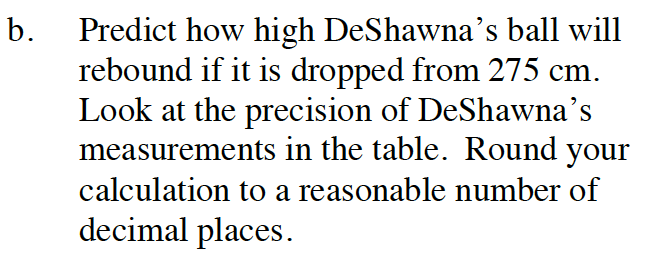Multiply the drop height by the ratio.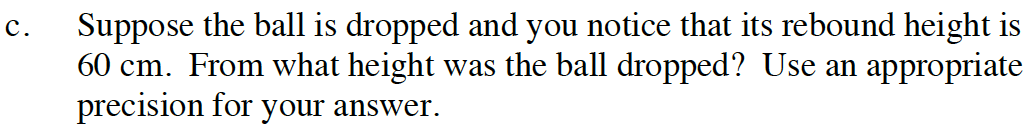Divide the rebound height by the ratio.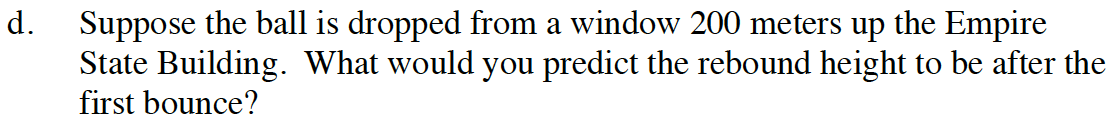See part (b).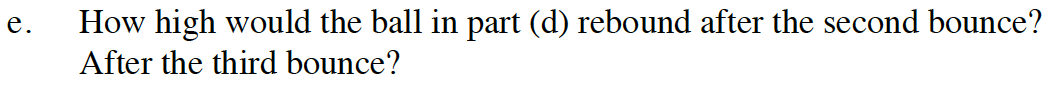Continue multiplying the drop height by the ratio to find the next rebound heights.